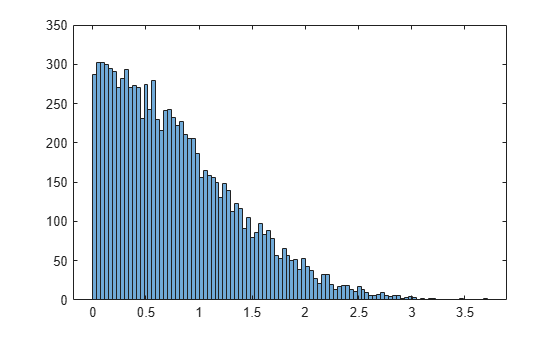# truncate

Truncate probability distribution object

## Syntax

``t = truncate(pd,lower,upper)``

## Description

example

````t = truncate(pd,lower,upper)` returns a probability distribution `t`, which is the probability distribution `pd` truncated to the specified interval with lower limit, `lower`, and upper limit, `upper`.```

## Examples

collapse all

Create a standard normal probability distribution object.

`pd = makedist('Normal')`
```pd = NormalDistribution Normal distribution mu = 0 sigma = 1 ```

Truncate the distribution to have a lower limit of -2 and an upper limit of 2.

`t = truncate(pd,-2,2)`
```t = NormalDistribution Normal distribution mu = 0 sigma = 1 Truncated to the interval [-2, 2] ```

Plot the pdf of the original and truncated distributions for a visual comparison.

```x = linspace(-3,3,1000); figure plot(x,pdf(pd,x)) hold on plot(x,pdf(t,x),'LineStyle','--') legend('Normal','Truncated') hold off```Create a standard normal probability distribution object.

`pd = makedist('Normal')`
```pd = NormalDistribution Normal distribution mu = 0 sigma = 1 ```

Truncate the distribution by restricting it to positive values. Set the lower limit to 0 and the upper limit to infinity.

`t = truncate(pd,0,inf)`
```t = NormalDistribution Normal distribution mu = 0 sigma = 1 Truncated to the interval [0, Inf] ```

Generate random numbers from the truncated distribution and visualize with a histogram.

```r = random(t,10000,1); histogram(r,100)```## Input Arguments

collapse all

Probability distribution, specified as a probability distribution object created using one of the following.

Function or AppDescription
`makedist`Create a probability distribution object using specified parameter values.
`fitdist`Fit a probability distribution object to sample data.
Distribution FitterFit a probability distribution to sample data using the interactive Distribution Fitter app and export the fitted object to the workspace.

Lower truncation limit, specified as a scalar value.

Data Types: `single` | `double`

Upper truncation limit, specified as a scalar value.

Data Types: `single` | `double`

## Output Arguments

collapse all

Truncated distribution, returned as a probability distribution object. The probability distribution function (pdf) of `t` is 0 outside the truncation interval. Inside the truncation interval, the pdf of `t` is equal to the pdf of `pd`, but divided by the probability assigned to that interval by `pd`.

The object properties of `t` are the same as those of `pd` with these exceptions:

• The `Truncation` property of `t` stores the truncation interval.

• The `IsTruncated` property of `t` is 1.

• The `InputData` property of `t` is empty. For a fitted distribution object, the `InputData` property stores the data used for distribution fitting. The truncated distribution object does not store the input data.